Related Articles
Menu driven program for all operations on doubly linked list in C
• Difficulty Level : Easy
• Last Updated : 27 Jan, 2021

A Linked List is a linear data structure that consists of two parts: one is the data part and the other is the address part. A Doubly Linked List in contains three parts: one is the data part and the other two are the address of the next and previous node in the list. In this article, all the common operations of a doubly linked list is discussed in one menu-driven program.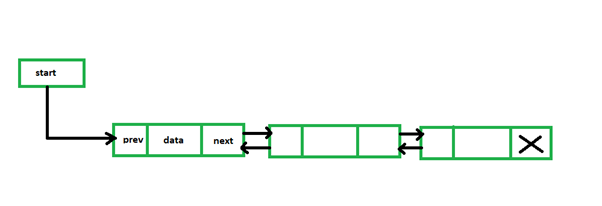Operations to be performed:

• traverse(): To see the contents of the linked list, it is necessary to traverse the given doubly linked list. The given traverse() function traverses and prints the content of the doubly linked list.
• insertAtFront(): This function simply inserts an element at the front/beginning of the doubly linked list.
• insertAtEnd(): This function inserts an element at the end of the doubly linked list.
• insertAtPosition(): This function inserts an element at a specified position in the doubly linked list.
• deleteFirst(): This function simply deletes an element from the front/beginning of the doubly linked list.
• deleteEnd(): This function simply deletes an element from the end of the doubly linked list.
• deletePosition(): This function deletes an element from a specified position in the doubly linked list.

Below is the implementation of the above operations:

## C

 `// C program for the all operations in``// the Doubly Linked List``#include ``#include `` ` `// Linked List Node``struct` `node {``    ``int` `info;``    ``struct` `node *prev, *next;``};``struct` `node* start = NULL;`` ` `// Function to traverse the linked list``void` `traverse()``{``    ``// List is empty``    ``if` `(start == NULL)``        ``printf``(``"\nList is empty\n"``);``    ``struct` `node* temp;`` ` `    ``// Else print the DLL``    ``else``    ``{``        ``temp = start;``        ``while` `(temp != NULL) {``            ``printf``(``"Data = %d\n"``,`` ``temp->info);``            ``temp = temp->next;``        ``}``    ``}``}`` ` `// Function to insert at the front``// of the linked list``void` `insertAtFront()``{``    ``int` `data;``    ``struct` `node* temp;``    ``temp = (``struct` `node*)``malloc``(``sizeof``(``struct` `node));``    ``printf``(``"\nEnter number to be inserted: "``);``    ``scanf``(``"%d"``, &data);``    ``temp->info = data;``    ``temp->prev = NULL;`` ` `    ``// Pointer of temp will be``    ``// assigned to start``    ``temp->next = start;``    ``start = temp;``}`` ` `// Function to insert at the end of``// the linked list``void` `insertAtEnd()``{``    ``int` `data;``    ``struct` `node *temp, *trav;``    ``temp = (``struct` `node*)``malloc``(``sizeof``(``struct` `node));``    ``temp->prev = NULL;``    ``temp->next = NULL;``    ``printf``(``"\nEnter number to be inserted: "``);``    ``scanf``(``"%d"``, &data);``    ``temp->info = data;``    ``temp->next = NULL;``    ``trav = start;`` ` `    ``// If start is NULL``    ``if` `(start == NULL) {`` ` `        ``start = temp;``    ``}`` ` `    ``// Changes Links``    ``else` `{``        ``while` `(trav->next != NULL)``            ``trav = trav->next;``        ``temp->prev = trav;``        ``trav->next = temp;``    ``}``}`` ` `// Function to insert at any specified``// position in the linked list``void` `insertAtPosition()``{``    ``int` `data, pos, i = 1;``    ``struct` `node *temp, *newnode;``    ``newnode = ``malloc``(``sizeof``(``struct` `node));``    ``newnode->next = NULL;``    ``newnode->prev = NULL;`` ` `    ``// Enter the position and data``    ``printf``(``"\nEnter position : "``);``    ``scanf``(``"%d"``, &pos);``    ``printf``(``"\nEnter number to be inserted: "``);``    ``scanf``(``"%d"``, &data);``    ``newnode->info = data;``    ``temp = start;`` ` `    ``// If start==NULL,``    ``if` `(start == NULL) {``        ``start = newnode;``        ``newnode->prev = NULL;``        ``newnode->next = NULL;``    ``}`` ` `    ``// If position==1,``    ``else` `if` `(pos == 1) {``        ``newnode->next = start;``        ``newnode->next->prev = newnode;``        ``newnode->prev = NULL;``        ``start = newnode;``    ``}`` ` `    ``// Change links``    ``else` `{``        ``while` `(i < pos - 1) {``            ``temp = temp->next;``            ``i++;``        ``}``        ``newnode->next = temp->next;``        ``newnode->prev = temp;``        ``temp->next = newnode;``        ``temp->next->prev = newnode;``    ``}``}`` ` `// Function to delete from the front``// of the linked list``void` `deleteFirst()``{``    ``struct` `node* temp;``    ``if` `(start == NULL)``        ``printf``(``"\nList is empty\n"``);``    ``else` `{``        ``temp = start;``        ``start = start->next;``        ``if` `(start != NULL)``            ``start->prev = NULL;``        ``free``(temp);``    ``}``}`` ` `// Function to delete from the end``// of the linked list``void` `deleteEnd()``{``    ``struct` `node* temp;``    ``if` `(start == NULL)``        ``printf``(``"\nList is empty\n"``);``    ``temp = start;``    ``while` `(temp->next != NULL)``        ``temp = temp->next;``    ``if` `(start->next == NULL)``        ``start = NULL;``    ``else` `{``        ``temp->prev->next = NULL;``        ``free``(temp);``    ``}``}`` ` `// Function to delete from any specified``// position from the linked list``void` `deletePosition()``{``    ``int` `pos, i = 1;``    ``struct` `node *temp, *position;``    ``temp = start;`` ` `    ``// If DLL is empty``    ``if` `(start == NULL)``        ``printf``(``"\nList is empty\n"``);`` ` `    ``// Otherwise``    ``else` `{``        ``// Position to be deleted``        ``printf``(``"\nEnter position : "``);``        ``scanf``(``"%d"``, &pos);`` ` `        ``// If the position is the first node``        ``if` `(pos == 1) {``            ``position = start;``            ``start = start->next;``            ``if` `(start != NULL) {``                ``start->prev = NULL;``            ``}``            ``free``(position);``            ``return``;``        ``}`` ` `        ``// Traverse till position``        ``while` `(i < pos - 1) {``            ``temp = temp->next;``            ``i++;``        ``}``        ``// Change Links``        ``position = temp->next;``        ``if` `(position->next != NULL)``            ``position->next->prev = temp;``        ``temp->next = position->next;`` ` `        ``// Free memory``        ``free``(position);``    ``}``}`` ` `// Driver Code``int` `main()``{``    ``int` `choice;``    ``while` `(1) {`` ` `        ``printf``(``"\n\t1  To see list\n"``);``        ``printf``(``"\t2  For insertion at"``               ``" starting\n"``);``        ``printf``(``"\t3  For insertion at"``               ``" end\n"``);``        ``printf``(``"\t4  For insertion at "``               ``"any position\n"``);``        ``printf``(``"\t5  For deletion of "``               ``"first element\n"``);``        ``printf``(``"\t6  For deletion of "``               ``"last element\n"``);``        ``printf``(``"\t7  For deletion of "``               ``"element at any position\n"``);``        ``printf``(``"\t8 To exit\n"``);``        ``printf``(``"\nEnter Choice :\n"``);``        ``scanf``(``"%d"``, &choice);`` ` `        ``switch` `(choice) {``        ``case` `1:``            ``traverse();``            ``break``;``        ``case` `2:``            ``insertAtFront();``            ``break``;``        ``case` `3:``            ``insertAtEnd();``            ``break``;``        ``case` `4:``            ``insertAtPosition();``            ``break``;``        ``case` `5:``            ``deleteFirst();``            ``break``;``        ``case` `6:``            ``deleteEnd();``            ``break``;``        ``case` `7:``            ``deletePosition();``            ``break``;`` ` `        ``case` `8:``            ``exit``(1);``            ``break``;``        ``default``:``            ``printf``(``"Incorrect Choice\n"``);``        ``}``    ``}``    ``return` `0;``}`

Output:

Menu: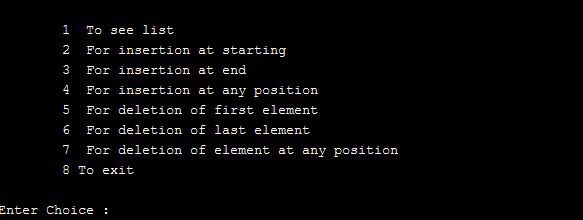Insertion at the starting: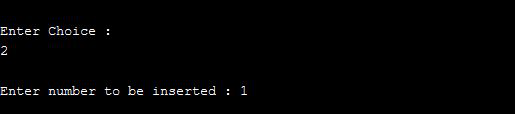Insertion at the end: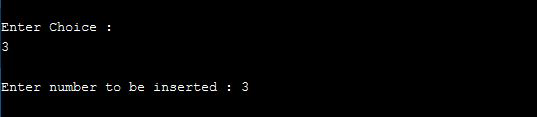Insertion at specific position: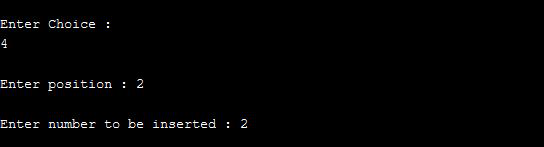Print the Linked List: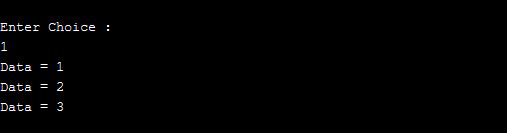Delete the first and last element with choice 5 and 6: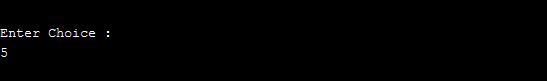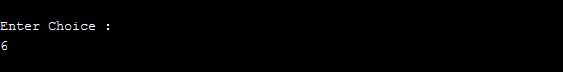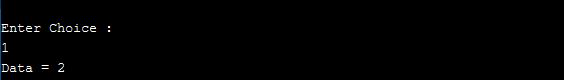Want to learn from the best curated videos and practice problems, check out the C Foundation Course for Basic to Advanced C.

My Personal Notes arrow_drop_up